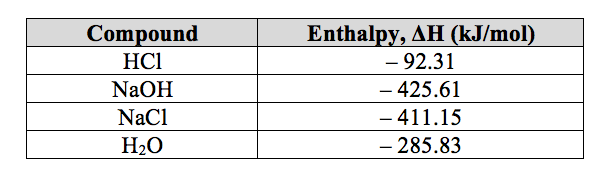# Problem: Calculate the standard enthalpy change for the following reaction at 25 °C. Standard enthalpy of formation values can be found in this table. HCl(g) + NaOH(s) → NaCl(s) + H 2O(l)

###### FREE Expert Solution###### Problem Details

Calculate the standard enthalpy change for the following reaction at 25 °C. Standard enthalpy of formation values can be found in this table.

HCl(g) + NaOH(s) → NaCl(s) + H 2O(l)What scientific concept do you need to know in order to solve this problem?

Our tutors have indicated that to solve this problem you will need to apply the Enthalpy of Formation concept. You can view video lessons to learn Enthalpy of Formation. Or if you need more Enthalpy of Formation practice, you can also practice Enthalpy of Formation practice problems.

What is the difficulty of this problem?

Our tutors rated the difficulty ofCalculate the standard enthalpy change for the following rea...as medium difficulty.

How long does this problem take to solve?

Our expert Chemistry tutor, Sabrina took 5 minutes and 25 seconds to solve this problem. You can follow their steps in the video explanation above.

What professor is this problem relevant for?

Based on our data, we think this problem is relevant for Professor Powe's class at UOFL.